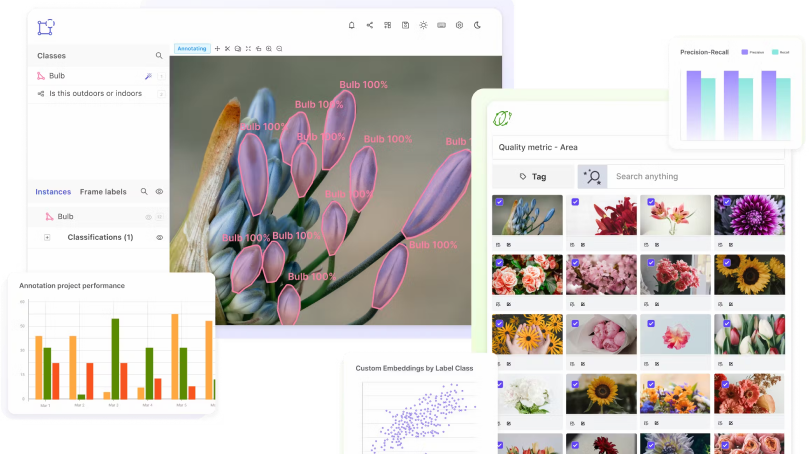# Precision

Encord Computer Vision Glossary

## Precision

Precision is a measure of the accuracy of a classifier or predictor in machine learning (ML). It is defined as the ratio of the number of true positive predictions made by the classifier to the total number of positive predictions made by the classifier. In other words, it is the proportion of positive predictions that are actually correct.

Precision is a crucial parameter in machine learning since it quantifies how well the classifier can recognise successful samples. It is sometimes combined with another statistic termed recall, which is defined as the proportion of true positive predictions to all cases that are in fact positive.

Precision and recall are trade-offs; for example, a classifier's recall may decline as its precision rises, and vice versa. The threshold parameter, which establishes the minimal probability necessary for a prediction to be deemed accurate, can be used to manage this trade-off.

Overall, precision is a measure of the accuracy of a classifier or predictor in ML, and is defined as the ratio of the number of true positive predictions to the total number of positive predictions. It is an important metric, and is often used in conjunction with recall to evaluate the performance of a classifier.

Scale your annotation workflows and power your model performance with data-driven insights## What is precision in machine learning?

Precision is a crucial parameter in machine learning since it quantifies how well the classifier can recognise successful samples. It is sometimes combined with another statistic termed recall, which is defined as the proportion of true positive predictions to all cases that are in fact positive.

Precision and recall are trade-offs; for example, a classifier's recall may decline as its precision rises, and vice versa. The threshold parameter, which establishes the minimal probability necessary for a prediction to be deemed accurate, can be used to manage this trade-off.

Overall, precision is a measure of the accuracy of a classifier or predictor in ML, and is defined as the ratio of the number of true positive predictions to the total number of positive predictions. It is an important metric, and is often used in conjunction with recall to evaluate the performance of a classifier.

#### The Complete Data Engine for AI Model Development###### Subscribe